# مجموعة (رياضيات)

(تم التحويل من جماعة)

في الرياضيات، المجموعة Group هي من اهم اسس و مواضيع الرياضيات التجريدية . اذا أردنا تعريف مبدئي يمكن القول أن كل وحدة تضم أشياء أو عناصر من العالم المادي أو غير المادي، الواقعي أو الخيالي تسمى مجموعة.

يمكن للزمرة ان تكون خالية و لكن لا يمكن لها ان تحتوي على نفس العنصر اكثر من مرة.

. . . . . . . . . . . . . . . . . . . . . . . . . . . . . . . . . . . . . . . . . . . . . . . . . . . . . . . . . . . . . . . . . . . . . . . . . . . . . . . . . . . . . . . . . . . . . . . . . . . . . . . . . . . . . . . . . . . . . . . . . . . . . . . . . . . . . . . . . . . . . . . . . . . . . . . . . . . . . . . . . . . . . . . .

## التعريف والتوضيح

### المثال الأول: الأعداد الصحيحة

One of the most familiar groups is the set of integers $\mathbb {Z}$which consists of the numbers

..., −4, −3, −2, −1, 0, 1, 2, 3, 4, ..., together with addition.

The following properties of integer addition serve as a model for the group axioms given in the definition below.

• For any two integers a and b, the sum a + b is also an integer. That is, addition of integers always yields an integer. This property is known as closure under addition.
• For all integers a, b and c, (a + b) + c = a + (b + c). Expressed in words, adding a to b first, and then adding the result to c gives the same final result as adding a to the sum of b and c, a property known as associativity.
• If a is any integer, then 0 + a = a + 0 = a. Zero is called the identity element of addition because adding it to any integer returns the same integer.
• For every integer a, there is an integer b such that a + b = b + a = 0. The integer b is called the inverse element of the integer a and is denoted −a.

The integers, together with the operation +, form a mathematical object belonging to a broad class sharing similar structural aspects. To appropriately understand these structures as a collective, the following definition is developed.

### Definition

The axioms for a group are short and natural... Yet somehow hidden behind these axioms is the monster simple group, a huge and extraordinary mathematical object, which appears to rely on numerous bizarre coincidences to exist. The axioms for groups give no obvious hint that anything like this exists.

Richard Borcherds in Mathematicians: An Outer View of the Inner World 

A group is a set, G, together with an operation ⋅ (called the group law of G) that combines any two elements a and b to form another element, denoted ab or ab. To qualify as a group, the set and operation, (G, ⋅), must satisfy four requirements known as the group axioms:

Closure
For all a, b in G, the result of the operation, ab, is also in G.b[›]
Associativity
For all a, b and c in G, (ab) ⋅ c = a ⋅ (bc).
Identity element
There exists an element e in G such that, for every element a in G, the equation ea = ae = a holds. Such an element is unique (see below), and thus one speaks of the identity element.
Inverse element
For each a in G, there exists an element b in G, commonly denoted a−1 (or −a, if the operation is denoted "+"), such that ab = ba = e, where e is the identity element.

The result of the group operation may depend on the order of the operands. In other words, the result of combining element a with element b need not yield the same result as combining element b with element a; the equation

ab = ba

may not be true for every two elements a and b. This equation always holds in the group of integers under addition, because a + b = b + a for any two integers (commutativity of addition). Groups for which the commutativity equation ab = ba always holds are called abelian groups (in honor of Niels Henrik Abel). The symmetry group described in the following section is an example of a group that is not abelian.

The identity element of a group G is often written as 1 or 1G, a notation inherited from the multiplicative identity. If a group is abelian, then one may choose to denote the group operation by + and the identity element by 0; in that case, the group is called an additive group. The identity element can also be written as id.

The set G is called the underlying set of the group (G, ⋅). Often the group's underlying set G is used as a short name for the group (G, ⋅). Along the same lines, shorthand expressions such as "a subset of the group G" or "an element of group G" are used when what is actually meant is "a subset of the underlying set G of the group (G, ⋅)" or "an element of the underlying set G of the group (G, ⋅)". Usually, it is clear from the context whether a symbol like G refers to a group or to an underlying set.

An alternate (but equivalent) definition is to expand the structure of a group to define a group as a set equipped with three operations satisfying the same axioms as above, with the "there exists" part removed in the two last axioms, these operations being the group law, as above, which is a binary operation, the inverse operation, which is a unary operation and maps a to $a^{-1},$and the identity element, which is viewed as a 0-ary operation.

As this formulation of the definition avoids existential quantifiers, it is generally preferred for computing with groups and for computer-aided proofs. This formulation exhibits groups as a variety of universal algebra. It is also useful for talking of properties of the inverse operation, as needed for defining topological groups and group objects.

### Second example: a symmetry group

Two figures in the plane are congruent if one can be changed into the other using a combination of rotations, reflections, and translations. Any figure is congruent to itself. However, some figures are congruent to themselves in more than one way, and these extra congruences are called symmetries. A square has eight symmetries. These are: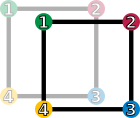id (keeping it as it is)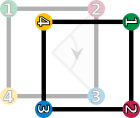r1 (rotation by 90° clockwise)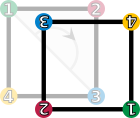r2 (rotation by 180° clockwise)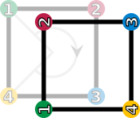r3 (rotation by 270° clockwise)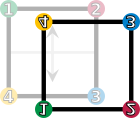fv (vertical reflection)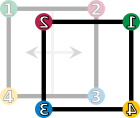fh (horizontal reflection)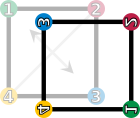fd (diagonal reflection)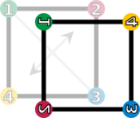fc (counter-diagonal reflection)
• the identity operation leaving everything unchanged, denoted id;
• rotations of the square around its center by 90° clockwise, 180° clockwise, and 270° clockwise, denoted by r1, r2 and r3, respectively;
• reflections about the horizontal and vertical middle line (fv and fh), or through the two diagonals (fd and fc).

These symmetries are functions. Each sends a point in the square to the corresponding point under the symmetry. For example, r1 sends a point to its rotation 90° clockwise around the square's center, and fh sends a point to its reflection across the square's vertical middle line. Composing two of these symmetries gives another symmetry. These symmetries determine a group called the dihedral group of degree 4, denoted D4. The underlying set of the group is the above set of symmetries, and the group operation is function composition. Two symmetries are combined by composing them as functions, that is, applying the first one to the square, and the second one to the result of the first application. The result of performing first a and then b is written symbolically from right to left as b ° a ("apply the symmetry b after performing the symmetry a"). (This is the usual notation for composition of functions.)

The group table on the right lists the results of all such compositions possible. For example, rotating by 270° clockwise (r3) and then reflecting horizontally (fh) is the same as performing a reflection along the diagonal (fd). Using the above symbols, highlighted in blue in the group table:

fh ∘ r3 = fd.
Group table of D4
id r1 r2 r3 fv fh fd fc
id id r1 r2 r3 fv fh fd fc
r1 r1 r2 r3 id fc fd fv fh
r2 r2 r3 id r1 fh fv fc fd
r3 r3 id r1 r2 fd fc fh fv
fv fv fd fh fc id r2 r1 r3
fh fh fc fv fd r2 id r3 r1
fd fd fh fc fv r3 r1 id r2
fc fc fv fd fh r1 r3 r2 id
The elements id, r1, r2, and r3 form a subgroup, highlighted in      red (upper left region). A left and right coset of this subgroup is highlighted in      green (in the last row) and      yellow (last column), respectively.

Given this set of symmetries and the described operation, the group axioms can be understood as follows:

1. The closure axiom demands that the composition ba of any two symmetries a and b is also a symmetry. Another example for the group operation is
r3 ∘ fh = fc,
i.e., rotating 270° clockwise after reflecting horizontally equals reflecting along the counter-diagonal (fc). Indeed every other combination of two symmetries still gives a symmetry, as can be checked using the group table.
2. The associativity constraint deals with composing more than two symmetries: Starting with three elements a, b and c of D4, there are two possible ways of using these three symmetries in this order to determine a symmetry of the square. One of these ways is to first compose a and b into a single symmetry, then to compose that symmetry with c. The other way is to first compose b and c, then to compose the resulting symmetry with a. The associativity condition
(ab) ∘ c = a ∘ (bc)
means that these two ways are the same, i.e., a product of many group elements can be simplified in any grouping. For example, (fd ∘ fv) ∘ r2 = fd ∘ (fv ∘ r2) can be checked using the group table at the right
 (fd ∘ fv) ∘∘ r2 = r3 ∘ r2 = r1, which equals fd ∘ (fv ∘ r2) = fd ∘ fh = r1.
While associativity is true for the symmetries of the square and addition of numbers, it is not true for all operations. For instance, subtraction of numbers is not associative: (7 − 3) − 2 = 2 is not the same as 7 − (3 − 2) = 6.
3. The identity element is the symmetry id leaving everything unchanged: for any symmetry a, performing id after a (or a after id) equals a, in symbolic form,
id ∘ a = a,
a ∘ id = a.
4. An inverse element undoes the transformation of some other element. Every symmetry can be undone: each of the following transformations—identity id, the reflections fh, fv, fd, fc and the 180° rotation r2—is its own inverse, because performing it twice brings the square back to its original orientation. The rotations r3 and r1 are each other's inverses, because rotating 90° and then rotation 270° (or vice versa) yields a rotation over 360° which leaves the square unchanged. In symbols,
fh ∘ fh = id,
r3 ∘ r1 = r1 ∘ r3 = id.

In contrast to the group of integers above, where the order of the operation is irrelevant, it does matter in D4, as, for example, fh ∘ r1 = fc but r1 ∘ fh = fd. In other words, D4 is not abelian, which makes the group structure more difficult than the integers introduced first. . . . . . . . . . . . . . . . . . . . . . . . . . . . . . . . . . . . . . . . . . . . . . . . . . . . . . . . . . . . . . . . . . . . . . . . . . . . . . . . . . . . . . . . . . . . . . . . . . . . . . . . . . . . . . . . . . . . . . . . . . . . . . . . . . . . . . . . . . . . . . . . . . . . . . . . . . . . . . . . . . . . . . . . .

## نتائج مباشرة

تعريف المجموعة يقود إلى عدد من النتائج المباشرة:

1. لا يوجد مجموعتين مختلفتين تضمان نفس العناصر.
2. يوجد مجموعات تضم مجموعات كعناصر.
3. المجموعة الخالية هي مجموعة جزئية من كل مجموعة.

## المجموعة الجزئية

إذا كان كل عنصر في المجموعة أ عنصرا في المجموعة ب تسمى عندها المجموعة أ مجموعة جزئية من ب. إذا كانت أ مجموعة جزئية من ب و ب مجموعة جزئية من أ ، عندها يكون أ=ب.

## أمثلة وتطبيقات

The fundamental group of a plane minus a point (bold) consists of loops around the missing point. This group is isomorphic to the integers.

Examples and applications of groups abound. A starting point is the group Z of integers with addition as group operation, introduced above. If instead of addition multiplication is considered, one obtains multiplicative groups. These groups are predecessors of important constructions in abstract algebra.

Groups are also applied in many other mathematical areas. Mathematical objects are often examined by associating groups to them and studying the properties of the corresponding groups. For example, Henri Poincaré founded what is now called algebraic topology by introducing the fundamental group. By means of this connection, topological properties such as proximity and continuity translate into properties of groups.i[›] For example, elements of the fundamental group are represented by loops. The second image at the right shows some loops in a plane minus a point. The blue loop is considered null-homotopic (and thus irrelevant), because it can be continuously shrunk to a point. The presence of the hole prevents the orange loop from being shrunk to a point. The fundamental group of the plane with a point deleted turns out to be infinite cyclic, generated by the orange loop (or any other loop winding once around the hole). This way, the fundamental group detects the hole.

In more recent applications, the influence has also been reversed to motivate geometric constructions by a group-theoretical background.j[›] In a similar vein, geometric group theory employs geometric concepts, for example in the study of hyperbolic groups. Further branches crucially applying groups include algebraic geometry and number theory.

In addition to the above theoretical applications, many practical applications of groups exist. Cryptography relies on the combination of the abstract group theory approach together with algorithmical knowledge obtained in computational group theory, in particular when implemented for finite groups. Applications of group theory are not restricted to mathematics; sciences such as physics, chemistry and computer science benefit from the concept.

### Numbers

Many number systems, such as the integers and the rationals enjoy a naturally given group structure. In some cases, such as with the rationals, both addition and multiplication operations give rise to group structures. Such number systems are predecessors to more general algebraic structures known as rings and fields. Further abstract algebraic concepts such as modules, vector spaces and algebras also form groups.

#### Integers

The group of integers $\mathbb {Z}$under addition, denoted $\left(\mathbb {Z} ,+\right)$, has been described above. The integers, with the operation of multiplication instead of addition, $\left(\mathbb {Z} ,\cdot \right)$do not form a group. The closure, associativity and identity axioms are satisfied, but inverses do not exist: for example, a = 2 is an integer, but the only solution to the equation a · b = 1 in this case is b = 1/2, which is a rational number, but not an integer. Hence not every element of $\mathbb {Z}$has a (multiplicative) inverse.k[›]

#### Rationals

The desire for the existence of multiplicative inverses suggests considering fractions

${\frac {a}{b}}.$Fractions of integers (with b nonzero) are known as rational numbers.l[›] The set of all such irreducible fractions is commonly denoted $\mathbb {Q}$. There is still a minor obstacle for $\left(\mathbb {Q} ,\cdot \right)$, the rationals with multiplication, being a group: because the rational number 0 does not have a multiplicative inverse (i.e., there is no x such that x · 0 = 1), $\left(\mathbb {Q} ,\cdot \right)$is still not a group.

However, the set of all nonzero rational numbers $\mathbb {Q} \setminus \left\{0\right\}=\left\{q\in \mathbb {Q} \mid q\neq 0\right\}$does form an abelian group under multiplication, generally denoted $\mathbb {Q} ^{*}$.m[›] Associativity and identity element axioms follow from the properties of integers. The closure requirement still holds true after removing zero, because the product of two nonzero rationals is never zero. Finally, the inverse of a/b is b/a, therefore the axiom of the inverse element is satisfied.

The rational numbers (including 0) also form a group under addition. Intertwining addition and multiplication operations yields more complicated structures called rings and—if division is possible, such as in $\mathbb {Q}$fields, which occupy a central position in abstract algebra. Group theoretic arguments therefore underlie parts of the theory of those entities.n[›]

### Modular arithmetic

In modular arithmetic, two integers are added and then the sum is divided by a positive integer called the modulus. The result of modular addition is the remainder of that division. For any modulus, n, the set of integers from 0 to n − 1 forms a group under modular addition: the inverse of any element a is na, and 0 is the identity element. This is familiar from the addition of hours on the face of a clock: if the hour hand is on 9 and is advanced 4 hours, it ends up on 1, as shown at the right. This is expressed by saying that 9 + 4 equals 1 "modulo 12" or, in symbols,

9 + 4 ≡ 1 modulo 12.

The group of integers modulo n is written $\mathbb {Z} _{n}$or $\mathbb {Z} /n\mathbb {Z}$.

For any prime number p, there is also the multiplicative group of integers modulo p. Its elements are the integers 1 to p − 1. The group operation is multiplication modulo p. That is, the usual product is divided by p and the remainder of this division is the result of modular multiplication. For example, if p = 5, there are four group elements 1, 2, 3, 4. In this group, 4 · 4 = 1, because the usual product 16 is equivalent to 1, which divided by 5 yields a remainder of 1. for 5 divides 16 − 1 = 15, denoted

16 ≡ 1 (mod 5).

The primality of p ensures that the product of two integers neither of which is divisible by p is not divisible by p either, hence the indicated set of classes is closed under multiplication.o[›] The identity element is 1, as usual for a multiplicative group, and the associativity follows from the corresponding property of integers. Finally, the inverse element axiom requires that given an integer a not divisible by p, there exists an integer b such that

a · b ≡ 1 (mod p), i.e., p divides the difference a · b − 1.

The inverse b can be found by using Bézout's identity and the fact that the greatest common divisor gcd(a, p) equals 1. In the case p = 5 above, the inverse of 4 is 4, and the inverse of 3 is 2, as 3 · 2 = 6 ≡ 1 (mod 5). Hence all group axioms are fulfilled. Actually, this example is similar to $\left(\mathbb {Q} \setminus \left\{0\right\},\cdot \right)$above: it consists of exactly those elements in $\mathbb {Z} /p\mathbb {Z}$that have a multiplicative inverse. These groups are denoted Fp×. They are crucial to public-key cryptography.p[›]

. . . . . . . . . . . . . . . . . . . . . . . . . . . . . . . . . . . . . . . . . . . . . . . . . . . . . . . . . . . . . . . . . . . . . . . . . . . . . . . . . . . . . . . . . . . . . . . . . . . . . . . . . . . . . . . . . . . . . . . . . . . . . . . . . . . . . . . . . . . . . . . . . . . . . . . . . . . . . . . . . . . . . . . .

### Cyclic groups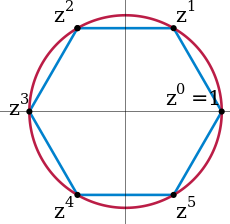The 6th complex roots of unity form a cyclic group. z is a primitive element, but z2 is not, because the odd powers of z are not a power of z2.

A cyclic group is a group all of whose elements are powers of a particular element a. In multiplicative notation, the elements of the group are:

..., a−3, a−2, a−1, a0 = e, a, a2, a3, ...,

where a2 means aa, and a−3 stands for a−1a−1a−1 = (aaa)−1 etc.h[›] Such an element a is called a generator or a primitive element of the group. In additive notation, the requirement for an element to be primitive is that each element of the group can be written as

..., −aa, −a, 0, a, a+a, ...

In the groups Z/nZ introduced above, the element 1 is primitive, so these groups are cyclic. Indeed, each element is expressible as a sum all of whose terms are 1. Any cyclic group with n elements is isomorphic to this group. A second example for cyclic groups is the group of n-th complex roots of unity, given by complex numbers z satisfying zn = 1. These numbers can be visualized as the vertices on a regular n-gon, as shown in blue at the right for n = 6. The group operation is multiplication of complex numbers. In the picture, multiplying with z corresponds to a counter-clockwise rotation by 60°. Using some field theory, the group Fp× can be shown to be cyclic: for example, if p = 5, 3 is a generator since 31 = 3, 32 = 9 ≡ 4, 33 ≡ 2, and 34 ≡ 1.

Some cyclic groups have an infinite number of elements. In these groups, for every non-zero element a, all the powers of a are distinct; despite the name "cyclic group", the powers of the elements do not cycle. An infinite cyclic group is isomorphic to (Z, +), the group of integers under addition introduced above. As these two prototypes are both abelian, so is any cyclic group.

The study of finitely generated abelian groups is quite mature, including the fundamental theorem of finitely generated abelian groups; and reflecting this state of affairs, many group-related notions, such as center and commutator, describe the extent to which a given group is not abelian.

### مجموعات التناظر

Symmetry groups are groups consisting of symmetries of given mathematical objects—be they of geometric nature, such as the introductory symmetry group of the square, or of algebraic nature, such as polynomial equations and their solutions. Conceptually, group theory can be thought of as the study of symmetry.t[›] Symmetries in mathematics greatly simplify the study of geometrical or analytical objects. A group is said to act on another mathematical object X if every group element performs some operation on X compatibly to the group law. In the rightmost example below, an element of order 7 of the (2,3,7) triangle group acts on the tiling by permuting the highlighted warped triangles (and the other ones, too). By a group action, the group pattern is connected to the structure of the object being acted on.

In chemical fields, such as crystallography, space groups and point groups describe molecular symmetries and crystal symmetries. These symmetries underlie the chemical and physical behavior of these systems, and group theory enables simplification of quantum mechanical analysis of these properties. For example, group theory is used to show that optical transitions between certain quantum levels cannot occur simply because of the symmetry of the states involved.

Not only are groups useful to assess the implications of symmetries in molecules, but surprisingly they also predict that molecules sometimes can change symmetry. The Jahn-Teller effect is a distortion of a molecule of high symmetry when it adopts a particular ground state of lower symmetry from a set of possible ground states that are related to each other by the symmetry operations of the molecule.

Likewise, group theory helps predict the changes in physical properties that occur when a material undergoes a phase transition, for example, from a cubic to a tetrahedral crystalline form. An example is ferroelectric materials, where the change from a paraelectric to a ferroelectric state occurs at the Curie temperature and is related to a change from the high-symmetry paraelectric state to the lower symmetry ferroelectric state, accompanied by a so-called soft phonon mode, a vibrational lattice mode that goes to zero frequency at the transition.

Such spontaneous symmetry breaking has found further application in elementary particle physics, where its occurrence is related to the appearance of Goldstone bosons.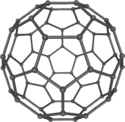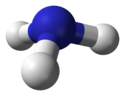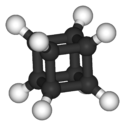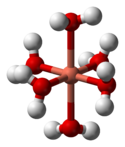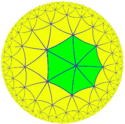Buckminsterfullerene displaysicosahedral symmetry, though the double bonds reduce this to pyritohedral symmetry. Ammonia, NH3. Its symmetry group is of order 6, generated by a 120° rotation and a reflection. Cubane C8H8 features octahedral symmetry. Hexaaquacopper(II) complex ion, [Cu(OH2)6]2+. Compared to a perfectly symmetrical shape, the molecule is vertically dilated by about 22% (Jahn-Teller effect). The (2,3,7) triangle group, a hyperbolic group, acts on this tiling of the hyperbolic plane.

Finite symmetry groups such as the Mathieu groups are used in coding theory, which is in turn applied in error correction of transmitted data, and in CD players. Another application is differential Galois theory, which characterizes functions having antiderivatives of a prescribed form, giving group-theoretic criteria for when solutions of certain differential equations are well-behaved.u[›] Geometric properties that remain stable under group actions are investigated in (geometric) invariant theory.

### General linear group and representation theory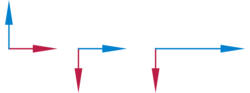Two vectors (the left illustration) multiplied by matrices (the middle and right illustrations). The middle illustration represents a clockwise rotation by 90°, while the right-most one stretches the x-coordinate by factor 2.

Matrix groups consist of matrices together with matrix multiplication. The general linear group GL(n, R) consists of all invertible n-by-n matrices with real entries. Its subgroups are referred to as matrix groups or linear groups. The dihedral group example mentioned above can be viewed as a (very small) matrix group. Another important matrix group is the special orthogonal group SO(n). It describes all possible rotations in n dimensions. Via Euler angles, rotation matrices are used in computer graphics.

## Generalizations

بنى شبيهة الزمرة
Totality Associativity حيادي Divisibility Commutativity
Semicategory غير مطلوب مطلوب غير مطلوب غير مطلوب غير مطلوب
Category غير مطلوب مطلوب مطلوب غير مطلوب غير مطلوب
Groupoid غير مطلوب مطلوب مطلوب مطلوب غير مطلوب
Magma مطلوب غير مطلوب غير مطلوب غير مطلوب غير مطلوب
Quasigroup مطلوب غير مطلوب غير مطلوب مطلوب غير مطلوب
Loop مطلوب غير مطلوب مطلوب مطلوب غير مطلوب
شبه زمرة مطلوب مطلوب غير مطلوب غير مطلوب غير مطلوب
مونويد مطلوب مطلوب مطلوب غير مطلوب غير مطلوب
زمرة مطلوب مطلوب مطلوب مطلوب غير مطلوب
زمرة أبيلية مطلوب مطلوب مطلوب مطلوب مطلوب
Closure, which is used in many sources, is an equivalent axiom to totality, though defined differently.

In abstract algebra, more general structures are defined by relaxing some of the axioms defining a group. For example, if the requirement that every element has an inverse is eliminated, the resulting algebraic structure is called a monoid. The natural numbers N (including 0) under addition form a monoid, as do the nonzero integers under multiplication (Z ∖ {0}, ·), see above. There is a general method to formally add inverses to elements to any (abelian) monoid, much the same way as (Q ∖ {0}, ·) is derived from (Z ∖ {0}, ·), known as the Grothendieck group. Groupoids are similar to groups except that the composition ab need not be defined for all a and b. They arise in the study of more complicated forms of symmetry, often in topological and analytical structures, such as the fundamental groupoid or stacks. Finally, it is possible to generalize any of these concepts by replacing the binary operation with an arbitrary n-ary one (i.e., an operation taking n arguments). With the proper generalization of the group axioms this gives rise to an n-ary group. The table gives a list of several structures generalizing groups.